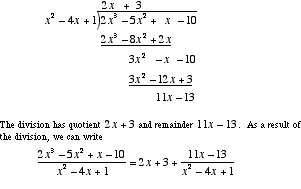index: click on a letter A B C D E F G H I J K L M N O P Q R S T U V W X Y Z A to Z index index: subject areas numbers & symbols sets, logic, proofs geometry algebra trigonometry advanced algebra & pre-calculus calculus advanced topics probability & statistics real world applications multimedia entrieswww.mathwords.com about mathwords website feedback

 Polynomial Long Division A method used to divide polynomials. Polynomial long division is essentially the same as long division for numbers. This method can be used to write an improper rational expression as the sum of a polynomial and a proper rational expression.See also Synthetic division